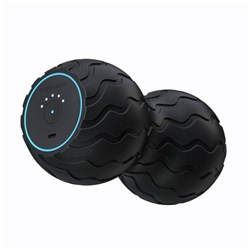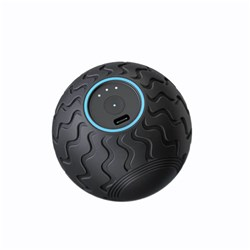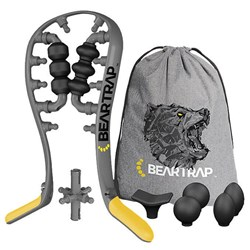# Massage & Trigger Points

Grid List
\$169.95
Increase value Decrease value
\$29.99
Increase value Decrease value
\$19.99
Increase value Decrease value
\$59.99
Increase value Decrease value
\$29.99
Increase value Decrease value
\$39.99
Increase value Decrease value
\$19.99
Increase value Decrease value
\$50.00
Increase value Decrease value
\$59.99
Increase value Decrease value
PTP Spinal Arch
In Stock
\$99.99
Increase value Decrease value
\$50.00
Increase value Decrease value
\$39.99
Increase value Decrease value
\$69.99
Increase value Decrease value
\$14.95
Increase value Decrease value
\$23.40
Increase value Decrease value
\$46.33
Increase value Decrease value
BakBalls Regular
In Stock
\$39.95
Increase value Decrease value
BakBalls Firm
In Stock
\$39.95
Increase value Decrease value
\$14.95
Increase value Decrease value
\$41.20
Increase value Decrease value
\$15.00
Increase value Decrease value\$99.00
Increase value Decrease value\$79.00
Increase value Decrease value
\$299.00
Increase value Decrease value
\$299.00
Increase value Decrease value
\$550.00
Increase value Decrease value
Beartrap Health
In Stock
\$299.00
Increase value Decrease value
\$39.95
Increase value Decrease value\$199.00
Increase value Decrease value
\$32.95
Increase value Decrease value
Jacknobber II
In Stock
\$31.59
Increase value Decrease value
\$35.20
Increase value Decrease value
\$17.00
Increase value Decrease value
\$11.99
Increase value Decrease value
\$181.50
Increase value Decrease value
\$50.30
Increase value Decrease value
\$187.00
Increase value Decrease value
\$12.99
Increase value Decrease value
\$149.00
Increase value Decrease value
\$525.00
Increase value Decrease value
\$10.95
Increase value Decrease value
\$14.55
Increase value Decrease value
RockTape RockPods Cupping Set
\$99.00
Increase value Decrease value
\$199.10
Increase value Decrease value
\$181.50
Increase value Decrease value
From
Increase value Decrease value
From
Increase value Decrease value
From
Increase value Decrease value
Grid List
##### Products to compare:
Comparing Products